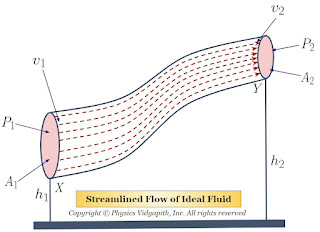## Necessity of Renewable or non-conventional energy sources

Renewable or non-conventional energy sources have become increasingly important in recent years due to several factors. Here are some of the key reasons why they are necessary:

Depletion of fossil fuels: Fossil fuels like coal, oil, and natural gas are finite resources that are being depleted at an alarming rate. As the demand for energy continues to grow, it is becoming more and more important to find alternative sources of energy that are sustainable and can be replenished.

Climate change: The use of fossil fuels is a major contributor to climate change, which is one of the greatest environmental challenges facing the world today. Renewable energy sources like solar, wind, and hydropower produce no greenhouse gas emissions and can help reduce the impact of climate change.

Energy security: Relying on imported oil and gas can leave a country vulnerable to supply disruptions and price fluctuations. By investing in renewable energy sources, countries can increase their energy security and reduce their dependence on foreign energy sources.

Economic benefits: Renewable energy sources are often cheaper in the long run than fossil fuels, especially as technology improves and economies of scale are achieved. Developing renewable energy industries can also create jobs and stimulate economic growth.

Energy access: In many parts of the world, access to energy is limited or nonexistent. Renewable energy sources like solar and wind power can be deployed quickly and affordably in remote areas, providing access to electricity for people who would otherwise have none.

In conclusion, the necessity of renewable or non-conventional energy sources is clear. They can help reduce our reliance on finite resources like fossil fuels, combat climate change, increase energy security, stimulate economic growth, and provide access to energy for people around the world. As technology continues to improve and costs come down, renewable energy sources will become an increasingly important part of our energy mix.

## Popular Posts

### Bernoulli's Theorem and Derivation of Bernoulli's EquationStatement of Bernoulli's Theorem: When an ideal fluid (i.e incompressible and non-viscous Liquid or Gas) flows in streamlined motion from one place to another, then the total energy per unit volume (i.e Pressure energy + Kinetic Energy + Potential Energy) at each and every of its path is constant. $P+\frac{1}{2}\rho v^{2} + \rho gh= constant$ Derivation of Bernoulli's Theorem Equation: Let us consider that an incompressible and non-viscous liquid is flowing in streamlined motion through a tube $XY$ of the non-uniform cross-section. Now Consider: The Area of cross-section $X$ = $A_{1}$ The Area of cross-section $Y$ = $A_{2}$ The velocity per second (i.e. equal to distance) of fluid at cross-section $X$ = $v_{1}$ The velocity per second (i.e. equal to distance) of fluid at cross-section $Y$ = $v_{2}$ The Pressure of fluid at cross-section $X$ = $P_{1}$ The Pressure of fluid at cross-section $Y$ = $P_{2}$ The height of cross-section $X$ from